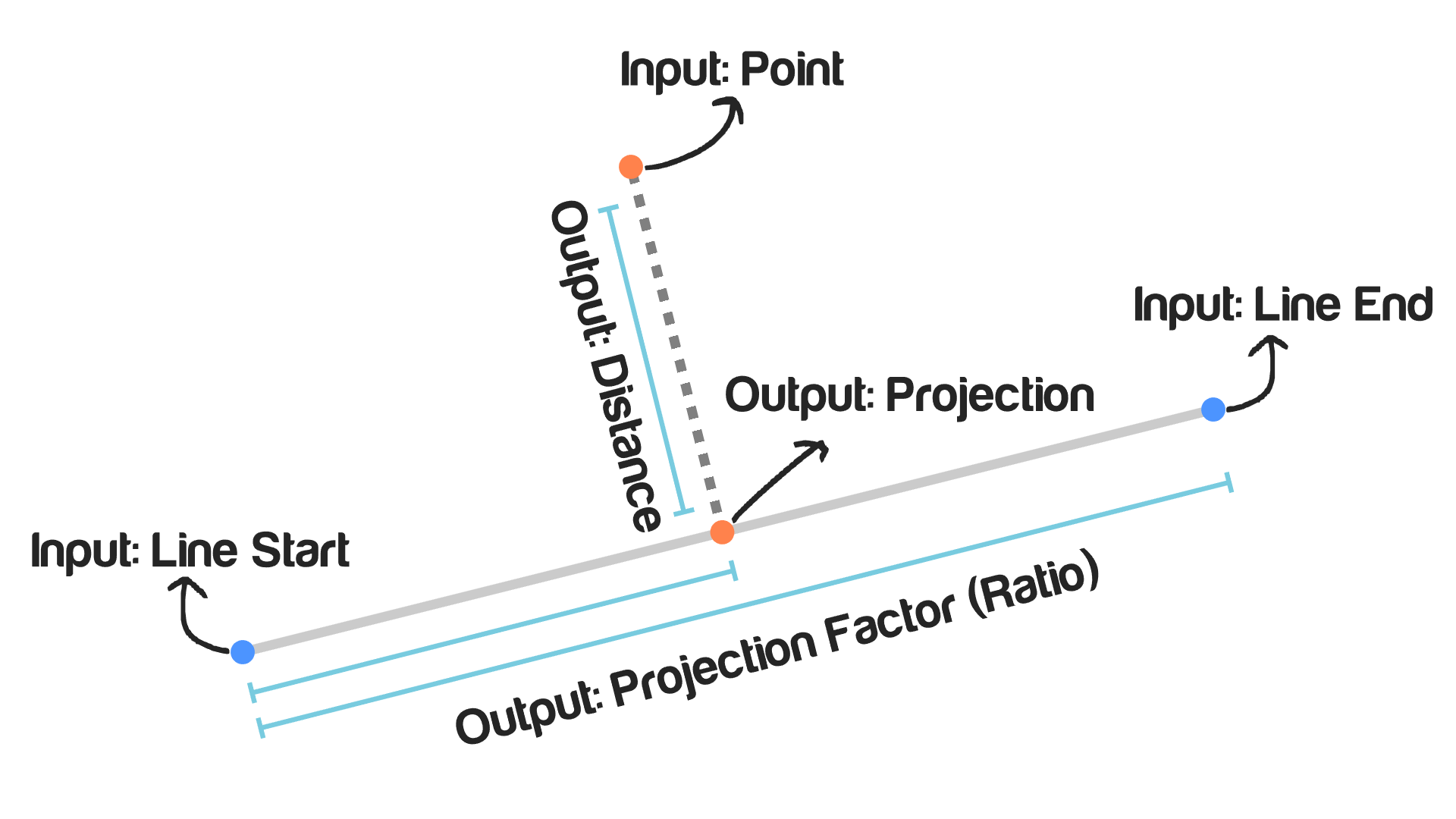# Project Point On Line

## Description

This node takes a point and a line and returns the projection of this point on that line. The projection of a point on a line is the closest point to the input point on the input line.

## Illustration## Inputs

• Point - The location of the point that will be projected.
• Line Start - The location of the line starting point.
• Line End - The location of the line ending point.

## Outputs

• Projection - The location of the closest point on the line to the input point. Mathematically this point is the point that makes a perpendicular line to the input line.
• Projection Factor - It is the ratio between the length of the line formed by the projected point and the input line’s first point and the length of the input line. Or in more mathematical word, it is the scalar of the convex combination of the starting and ending point to get the projected point.
• Distance - It is the shortest distance between the input point and the input line, which is also the distance between the projected point and the input point.